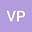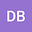loading page

Numerical solutions of electromagnetic wave model of fractional derivative using class of finite difference scheme
••Dhirendra Bahuguna
Indian Institute of Technology Kanpur
Author Profile## Abstract

In this article, a numerical scheme is introduced for solving the fractional partial differential equation (FPDE) arising from electromagnetic waves in dielectric media (EMWDM) by using an efficient class of finite difference methods. The numerical scheme is based on the Hermite formula. The Caputo’s fractional derivatives in time are discretized by a finite difference scheme of order O((k^(4-alpha)) & O(k^(4-beta)), 1

#### Peer review status:UNDER REVIEW

28 Feb 2021Submitted to Mathematical Methods in the Applied Sciences
28 Feb 2021Assigned to Editor
28 Feb 2021Submission Checks Completed
06 Mar 2021Reviewer(s) Assigned